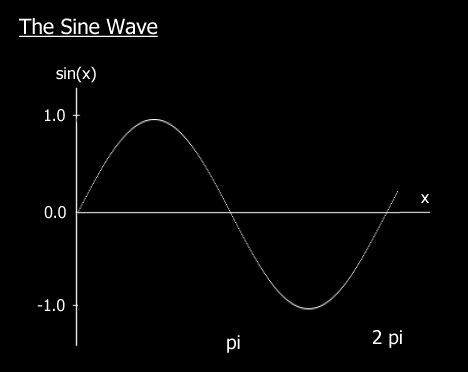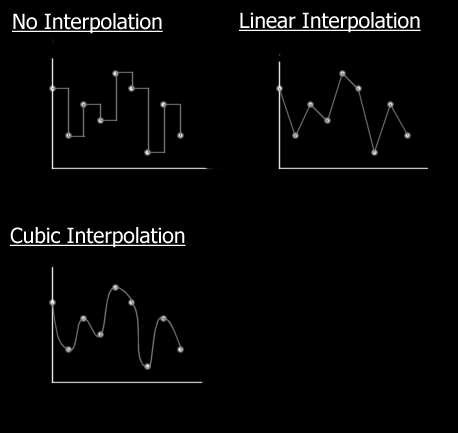The Art of Demomaking - Issue 03 - Timer Related Issues by (06 September 1999) Return to The Archives
 IntroductionThis week's tutorial focuses on very important techniques for demomaking and game programming, mainly involving speed related problems and synchronisation. I was going to talk about all this later in the series, but it's so important that I'd rather explain all these problems now.

 Timing DevicesUnlike Einstein's theory, time isn't relative to the speed of your PC :) And that's one of the main problems with programming for the PC platform: different machines in different conditions will run the program at different speeds. And as a demomaker or game developer these are problems which you must face to make your program look and behave the same way whatever.

One way of making sure of this is to have a variable that is incremented at regular intervals no matter what, just like a clock. All the calculations used to draw the frame must be of course based on that counter. That way the effect will look the same whatever the frame rate.

There are various platform dependant methods of getting such a variable. Under DOS you can access a double word variable in memory that always contains the current time, and that is updated 18.2 times per second. You can also hook the timer interrupt, which means you can create a procedure that will be called regularly. In Windows there is a function GetTickCount() that also returns the current time in a long integer. Under Linux and WinNT you can create a special thread which does the same thing as the DOS interrupt hook.

But how you get your timing device is irrelevant, the concept remains the same.

 ``` Main Procedure Set video mode Initialise the timing device Run loop Draw the effect given the current time Increase frame count Until a key is pressed Close up the timing device Return to old video mode Calculate frames per second (frame count / total time) End Main ```

For the next tutorials, we will need some timing device capable of doing this in DJGPP. It turns out there is a nice unit called TIME.H that does most of the work for us. We just need to wrap it all up into a clean C++ object, and we're in business :)

 Frames Per SecondAs most keen gamers will know, the FPS ratio is the average number of times a new image is displayed on the screen per second. High FPS, or at least above 30 is essential for game playability. But all the demos we will code will be non interactive. So how important is the FPS for us?To make an effect look the smoothest as possible, there should be a screen update for every screen refresh, that's about 60 FPS. In the old days of the amiga, demomakers would not release an effect unless it ran at one frame per retrace. Sure that takes a lot of skill and optimisation, but I seriously reckon that's a bit of a shame. I'd personally rather see an amazing effect at 20 FPS, than a good effect at 60 FPS. The fact that a demo is non interactive makes anything above 10 FPS acceptable. Anything below is not quite as enjoyable to watch.

 Dispelling the Myth of PrecalculationFixed Point MathThe advantage of floating point math is that you can work with reasonable precision at virtually any scale, but you pay a price for that: speed. Fixed point math works fine as long as you can put up with being limited to a certain range. The benefits being better execution time, like on the new Athlon which has 8 integer units against only 3 floating point units.

So, how does fixed point work i hear you ask... Simple! Suppose that instead of using a whole 16 bit word to store the integer part of a number, we split that up. We can assign 8 bits for the integer part, and obviously 8 bits for the fractional part. You can also split any variable as you like, but this method has many advantages, including the fact that it is simply split into 2 bytes.

So with this method, we can store a number with an integer part within the range -128..127 and with a fractional value that has 1/256th precision. Note that this method works just as well with unsigned numbers.Additionally, conversion from fixed point to floating point, and vice versa, is quite easy. A is a fixed point integer, and B is a float.

 ``` A = (int)( B * 256.0f ) B = (float)(A) / 256.0f ```

Note that 256.0f = 2^8, because we have a 8 bit fractional part.

Given those equations, basic binary operations on fixed point numbers are straight forward. Obviously C and D are also fixed point.

 ``` A = C + D A = C - D ```

For multiplication and division, things get a little tricky. If we just multiply two 8.8 fixed point numbers together, we get a 16.16 fixed point value. So we must shift 8 bits to the right to revert to 16.8, and then simply truncate to 8.8. For division, we'd end up with a 16 bit integer with no fractional part, so we must shift 8 bits to the left to introduce the fractional part again.

 ``` A = ( C * D ) >> 8 A = ( C << 8 ) / D ```

The best thing to do to convince yourself of the gain of speed is to convert the example later in this tutorial to floating point.

 InterpolationGiven a number of arbitrary values, interpolation is a technique that enables you to find as many intermediary values as you wish. The easiest way to think of interpolation is with a graph. You have a series of values, one for each integer within a certain range. Now you may want to find the value corresponding to any float within that range.Note that there are many different kinds of interpolation techniques, the cubic method being the nicest, but most time consuming. The method we will use, linear interpolation, has the advantage of being simple and quick, and good enough quality in most cases.

If you actually understand interpolation, programming it is the easy part. Say we have two values, A and B again, and we wish to find a intermediary value C. All we need is a blending coefficient k, which is always contained within the range 0..1. If the coefficient is 0, C has the value of A, and as k gets closer to 1, C becomes closer to B. This is translated by the mathematical equation:

 ` C = A + (B-A) * k `

You may notice that this equation is very similar to that of a line, hence the adjective linear describing the interpolation :)

 Cross FadingFinal words